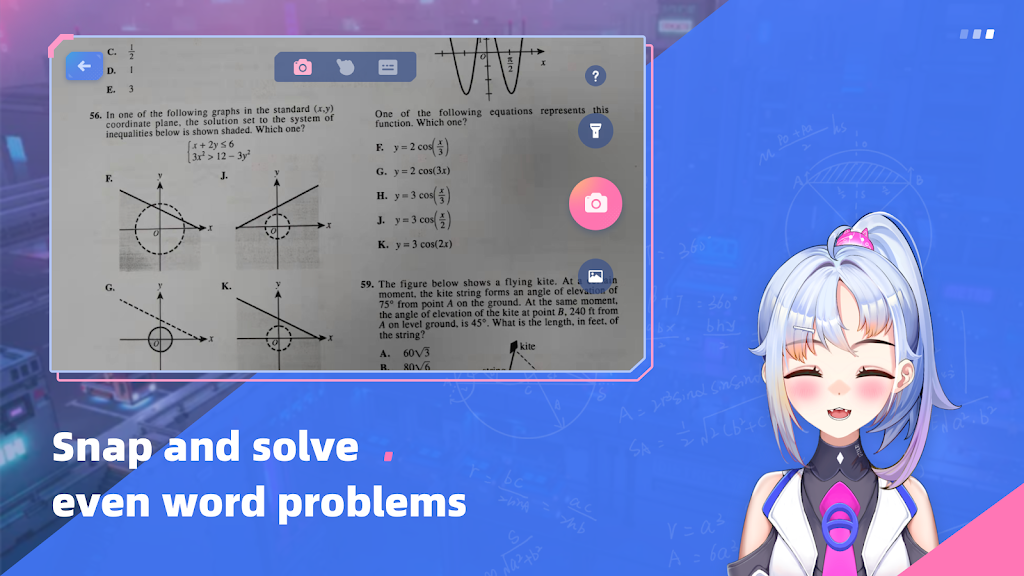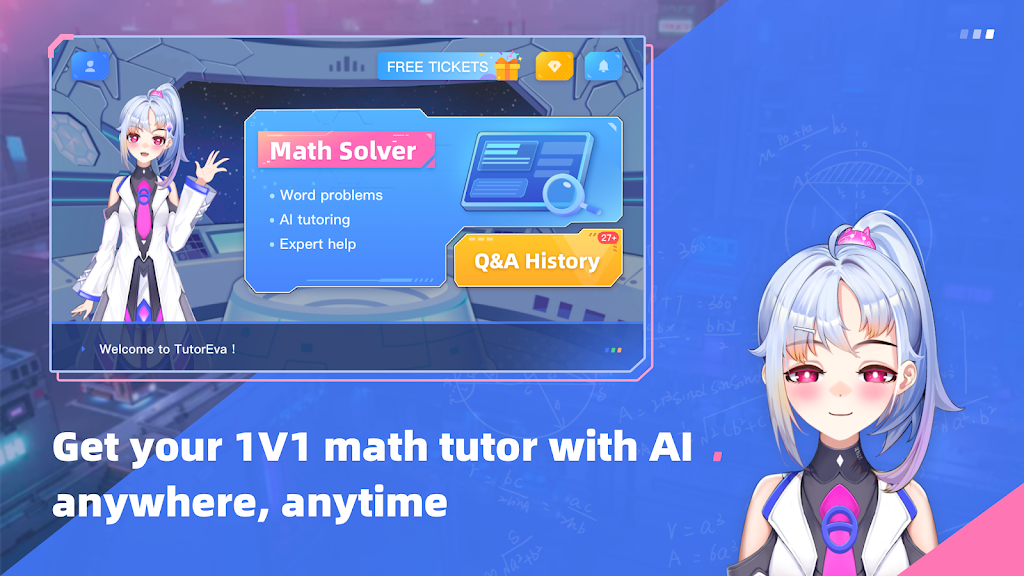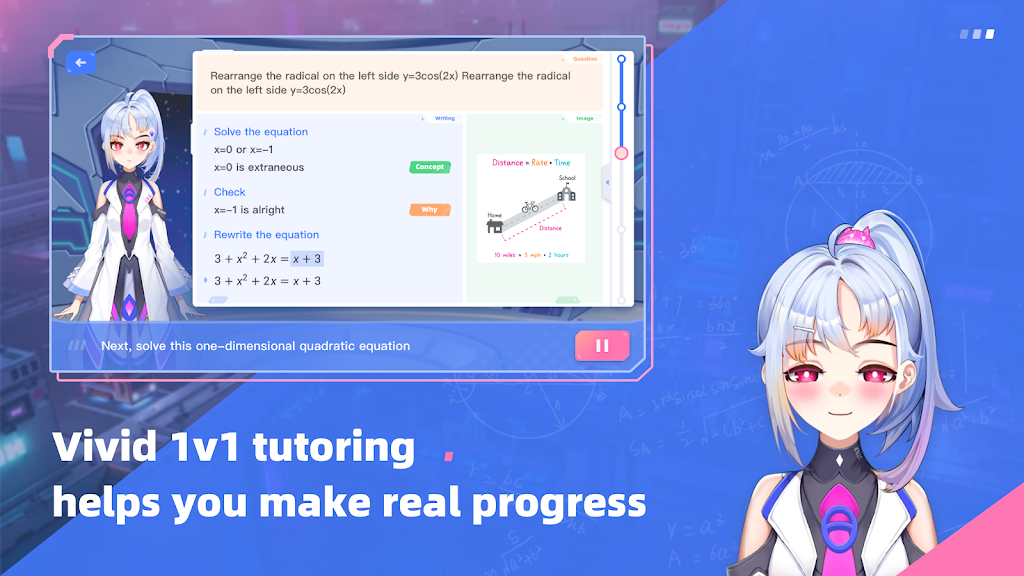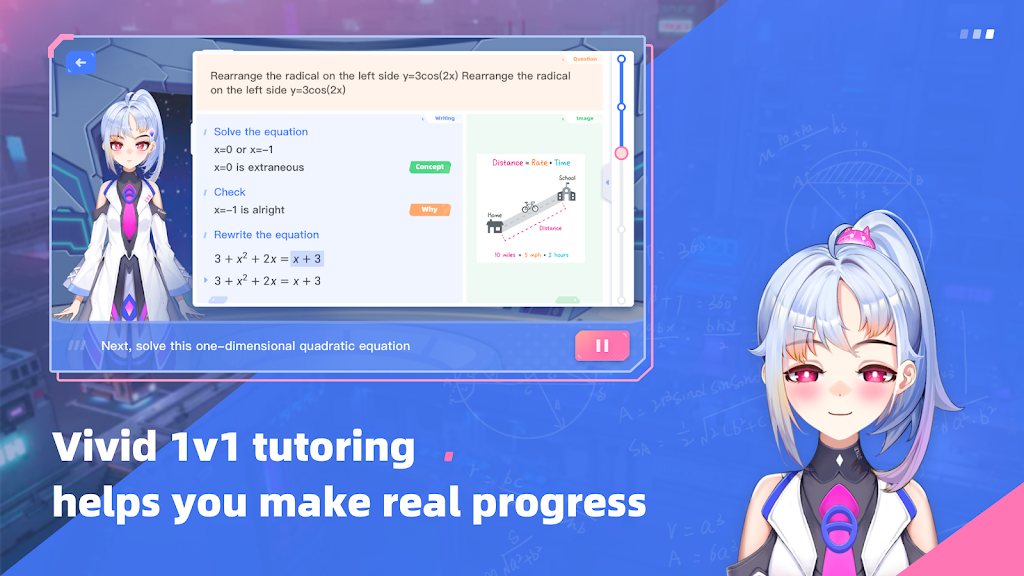-->

App By:
Version:
1.7.1 for Android
Updated On:
Sep 29, 2022
Size:
76 MB
Required Android:
Android 6.0
Category:
Educational

Have you ever imagined having your own AI math tutor?She solves all your math problems,

provides precise answers and step-by-step solutions.

She can even show you HOW to solve these problems,

in a vivid and interactive way,

like a real teacher.

she will respond,

and patiently explain in further detail.She will greet you with delight,

accompany you like a friend,

make your math trip fun and efficient.

This is what we are offering you.

We call her Eva,

Educational Virtual Tutor.and get your personal AI tutor now!

### KEY FEATURES:

• Solving almost all math problems
• Step-by-step solutions and problem-solving methods
• Learning through 1v1 interaction with AI tutor
• Communicative and fast responses
• Exquisive and fashionable AI avatar
• More than a math solver### MATH TOPICS COVERED：

• Basic Algebra: arithmetic operation, numerical factorization, algebraic operation , factorization, solve for equation(sets), solve for inequality(sets)
• Elementary function: analyzing elementary functions, graphing elementary functions , Function Operations (addition, subtraction, multiplication, division ) & Compositions
• Basic geometry: geometrical proof. Geometrical operation
• Analytic geometry: Circle, Conic section
• Math modeling: list algebraic expression(s) for word-problems, list equations or inequalities for word-problems, function applications, geometric applications, function applications
• Elementary statistics and probability: trigonometry , simple matrixes and vectors, simple series and sequences.
• Even hard word problems！

Artificial Intelligence tutor Eva is your personal tutor. For your math problems, even including obscure word problems, it cracks each problem in various ways, provides step-by-step solutions and presents targeted explanation, giving you the help exactly what you need.

With the company of EVA, doing math homework is no longer lonely and painful.